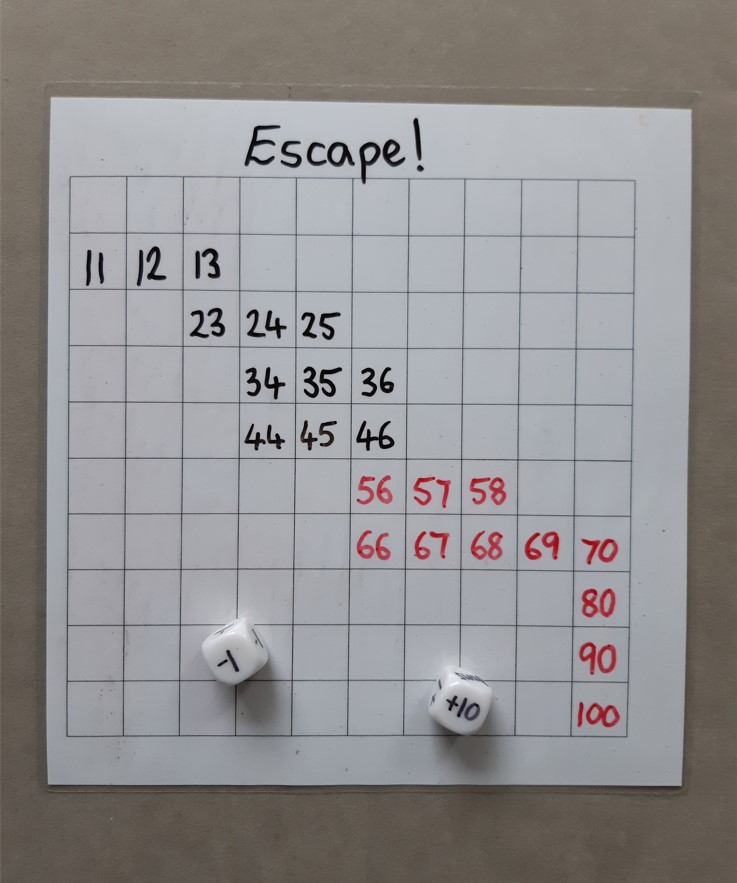## Escape!

A game for 2 players

The aim of this game is to escape the 100 square. It reinforces the place value of 10s and 1s through adding and subtracting 1 or 10.

### You will need:

• A 100 square
• Two small counters
• A 6-sided subtraction dice marked with -1, -1, -1, -10, -10, swap
• A 6-sided addition dice marked with +1, +1, +1, +10, +10, swap
• ### How to play

One player takes the subtraction dice and places their counter on number 45 on the 100 square. The other play takes the addition dice and places their counter on number 56 on the 100 square.

Players take turns to roll the dice and move their counter appropriately on the 100 square. When a player rolls ‘swap’, they must swap dice with their opponent, immediately rolling again. Players keep those dice until ‘swap’ is rolled again. If swap is rolled more than once on a players turn, keep swapping and rolling until a calculation is rolled!

The winner is the first person to make a move that takes them off the 100 square. If appropriate, the player may be challenged to say what number they would land on.

### Variations

• Use an empty 100 square and record the number you finish on each turn.
• Use a 100 square that starts on 101 (or 251 ), to practise adding and subtracting with 3-digit numbers. Put counters on 145 and 156 (or 295 and 306) to start.
• Use a decimal 100 square to practise adding tenths and hundredths. Put counters on 0.45 and 0.56 to start.
• Use a 100 square from -49 to 50 , to practise adding and subtracting from positive and negative numbers. Put counters on -5 and 6 to start.
• Put your own numbers into an empty 100 square!
•